## Example Questions

### Example Question #1 : Equilateral Triangles

What is the height of an equilateral triangle with a side length of 8 in?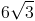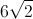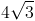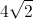Explanation:

An equilateral triangle has three congruent sides, and is also an equiangular triangle with three congruent angles that each meansure 60 degrees.

To find the height we divide the triangle into two special 30 - 60 - 90 right triangles by drawing a line from one corner to the center of the opposite side. This segment will be the height, and will be opposite from one of the 60 degree angles and adjacent to a 30 degree angle. The special right triangle gives side ratios of,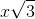, and. The hypoteneuse, the side opposite the 90 degree angle, is the full length of one side of the triangle and is equal to. Using this information, we can find the lengths of each side fo the special triangle.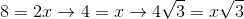The side with lengthwill be the height (opposite the 60 degree angle). The height is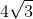inches.

### Example Question #1 : How To Find The Height Of An Equilateral Triangle

Find the height of a triangle if all sides have a length of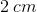.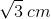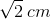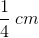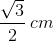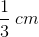Explanation:

Draw a vertical line from the vertex. This will divide the equilateral triangle into two congruent right triangles. For the hypothenuse of one right triangle, the length will be. The base will have a dimension of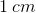.  Use the Pythagorean Theorem to solve for the height, substituting infor, the length of the hypotenuse, andfor eitheror, the length of the legs of the triangle: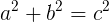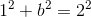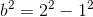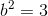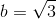### Example Question #2 : How To Find The Height Of An Equilateral Triangle

What is the height of an equilateral triangle with sides of length?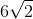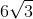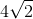Explanation:

Recall that from any vertex of an equilateral triangle, you can drop a height that is a bisector of that vertex as well as a bisector of the correlative side. This gives you the following figure: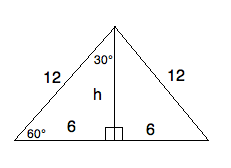Notice that the small triangles within the larger triangle are both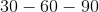triangles. Therefore, you can create a ratio to help you find.

The ratio oftois the same as the ratio ofto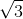.

As an equation, this is written: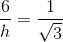Solving for, we get: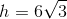### Example Question #3 : How To Find The Height Of An Equilateral Triangle

The Triangle Perpendicular Bisector Theorem states that the perpendicular bisector of an equilateral triangle is also the triangle's height.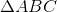is an equilateral triangle with side lengthinches. What is the height of?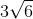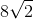Explanation:

To calculate the height of an equilateral triangle, first draw a perpendicular bisector for the triangle. By definition, this splits the opposite side from the vertex of the bisector in two, resulting in two line segments of lengthinches. Since it is perpendicular, we also know the angle of intersection isSo, we have a new right triangle with two side lenghtsandfor the hypotenuse and short leg, respectively. The Pythagorean theorem takes over from here: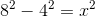--->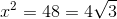So, the height of our triangle is.